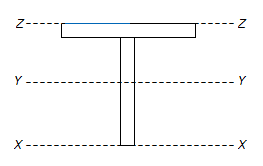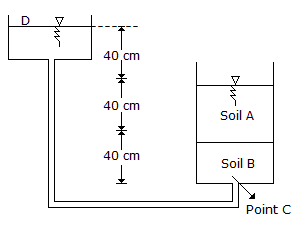# Online Civil Engineering Test - Previous GATE Exam Papers Test 4Loading Test...

Instruction:

• This is a FREE online test. DO NOT pay money to anyone to attend this test.
• Total number of questions : 20.
• Time alloted : 30 minutes.
• Each question carry 1 mark, no negative marks.
• DO NOT refresh the page.
• All the best :-).

1.

The permissible bending tensile stress in concrete for the vertical wall of an R.C. water tank made of M 25 concrete is :

A.
 8.5 N/mm2B.
 6.0 N/mm3C.
 2.5 N/mm2D.
 1.8 N/mm22.

The cylinder strength of the concrete is less than the cube strength because of

A.
 the difference in the shape of the cross section of the specimensB.
 the difference in the slendeness ratio of the specimensC.
 the friction between the concrete specimens and the steel plate of the testing machineD.
 the cubes are tested without capping but the cylinders are tested with capping3.

Vane tester is normally used for determining in situ shear strength of :

A.
 soft claysB.
 sandC.
 stiff claysD.
 gravel4.

The basic assumption of plane sections normal to the neutral axis before bending, remaining plane and normal to the neutral axis after bending, leads to :

A.
 uniform strain over the beam cross sectionB.
 uniform stress over the cross sectionC.
 linearly varying strain over the cross sectionD.
 stresses which are proportional to strains at all cross sections5.

Negative skin friction occurs when :

A.
 an upward drag exists in the pileB.
 the surrounding soil settles more than the pileC.
 the pile passes continuously through a firm soilD.
 the driving operation begins6.

Both Reynolds and Froude numbers assume significance in one of following examples :

A.
 Motion of submarine at large depthsB.
 Motion of ship in deep seasC.
 Cruising of a missile in airD.
 Flow over spillways7.

Two footings, one circular and the other square, are founded on the surface of a purely cohesionless soil. The diameter of the circular footing is same as that of the side of the square footing. The ratio of their ultimate bearing capcities is

A.
 3/4B.
 4/3C.
 1D.
 1.38.

Design rate of super elevation for horizontal highway curve of radius 450 m for a mixed traffic condition, having a speed of 125 km/hour is

A.
 1B.
 0.05C.
 0.07D.
 0.1549.

If soil is dried beyond its shrinkage limit, it will show :

A.
 Large volume changeB.
 Moderate volume changeC.
 Low volume changeD.
 No volume change10.

If YY is the centroidal axis of a T beam-section subjected to plastic moment, Mp, the neutral axis lies,A.
 above the line ZZB.
 between the lines YY and ZZC.
 between the lines XX and YYD.
 below the line XX11.

The main constituents of gas generated during the anaerobic digestion of sewage sludge are :

A.
 carbon dioxide and methaneB.
 methane and ethaneC.
 carbon dioxide and carbon monoxideD.
 carbon monoxide and nitrogen12.

Two samples of water A and B have pH values of 4.4 and 6.4 respectively. How many times more acidic sample A is than sample B ?

A.
 0B.
 50C.
 100D.
 20013.

Rapid curing cut back bitumen is produced by blending bitumen with

A.
 KeroseneB.
 BenzeneC.
 DieselD.
 Petrol14.

A river 5 m deep consists of a sand bed with saturated unit weight of 20 kN/m3 . vw = 9.81 kN/m3. The effective vertical stress at 5 m from the top of sand bed is

A.
 41 kN/m2B.
 51 kN/m2C.
 55 kN/m2D.
 53 kN/m215.

Delta (Δ) in cm. Duty (D) in hectare Cumec and Base period (B) in days are related as

A.
 Δ = 864 B/DB.
 B = 864 D/ΔC.
 B = 864 Δ/DD.
 D = 8.64 B/Δ16.

The Piezometric head at point C, in the experimental set-up shown in the below figure when the flow takes place under a constant head through the soils A and B isA.
 0 cmB.
 40 cmC.
 80 cmD.
 120 cm17.

A prestressed concrete rectangular beam of size 300 mm x 900 mm is prestressed with an initial prestressing force of 700 kN at an eccentricity of 350 mm at midspan. Stress at top of the beam due to prestress alone, in N/mm2 is

A.
 46 (tension)B.
 2.59 (compression)C.
 zeroD.
 8.64 (compression)18.

The type of surveying in which the curvature of the earth is taken into account is called

A.
 Geodetic surveyingB.
 Plane surveyingC.
 Preliminary surveyingD.
 Topographical surveying19.

Base course is used in rigid pavements for

A.
 prevention of subgrade settlementB.
 prevention of slab crackingC.
 prevention of pumpingD.
 prevention of thermal expansion20.

The ultimate bearing capacity of a soil is 300 kN/m2. The depth of foundation is 1 m and unit weight of soil is 20 kN/m3. Choosing a factor of safety of 2.5, the net safe bearing cacpacity is

A.
 100 kN/m2B.
 112 kN/m2C.
 800 kN/m2D.
 100.5 kN/m2